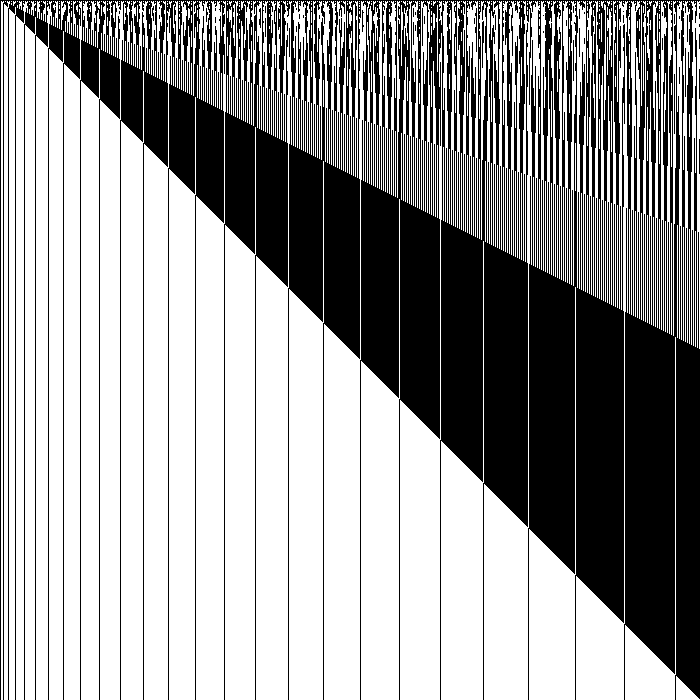# Lockers Problem (and Solution)

## Source

The inspiration for this entry came from a question posed on The Daily WTF. To paraphrase: there are 100 lockers, all closed, and one needs to 'toggle' (logical NOT - if it is open, close it, otherwise open it) each nth locker for n from 1 to 100. So for instance, first n=1 so toggle lockers (1,2,3,4...100) so all are open. Next n=2, so toggle (2,4,6,8...100) so all odd-numbered ones are open and all even-numbered ones closed. Then n=3 so toggle (3,6,9...99), etc, up to n=99 so toggle (99), and finally n=100 so toggle (100).

What lockers remain open after this is done? Brute force / counting methods are not allowed. I would say no programming allowed also.

## Solution

A possible way to arrive at a solution is described below (that was a spoiler alert).

It took me a while to get the basis of this problem. First I made a few observations:

• The operation is NOT, which is reversible and has only two states. By implication, the order in which the lockers are toggled thus does not matter. Toggling from n=100 to 1 will give the same result as n=1 to 100.
• The first run opens all the lockers, so can be ignored (instead set initial state to open)
• All runs for n>50 will only toggle one locker, so all of them (n=51 to 100) can be replaced by a single inversion operation on the upper half of the lockers.

Now it becomes more challenging. The problem is now reduced in half but still not obvious to solve. To move further towards abstracting the problem I began with a simpler system of 10 lockers and increased the number from there:

• If a solution is calculated for n lockers, adding another locker to the problem will not affect that solution. This is important as it means that the final state of each locker is dependent solely on its 'locker number' and not on the states of other lockers.
• Runs for two values of n with gcd(n1,n2)>1 will result in 'destructive interference' in that some of the lockers will not be affected. For instance, n=2 and n=4 results in the n=4 lockers returning to their original state.
• Combining the above facts, lockers with a 'locker number' that has an even number of factors (all unique factors, not just prime, including 1 and the locker number itself) will remain unaffected (closed), while those with an odd number of factors will end up being open at the end of the procedure.

This is sufficient grounds for a solution. If all unique factors, including 1 and the number itself are considered, what feature determines whether the number of factors is odd or even? It looks like this would be dependent on repeat factors - with two repeat factors for instance, one factor will not be included in the list thus making the total number of factors odd. For instance, factors of 4 are 1,2,4, while for 10 they are 1,2,5,10 since 4 has 2 as a repeat factor.

Now the problem is to generalize that description to a certain number set. By a proof that is left to the reader, it can be seen that this is true only for square numbers. Thus, lockers 1,4,9,16...81,100 will be open, while the rest will be closed (initial state).

## Programmatic Solution and Graph

To confirm the solution, I generated a brute-force program that displayed the results of each step of the algorithm performed on 700 lockers (note that since the number of lockers does not affect the solution set, this presents valid solutions for all variations of this problem from 1 to 700 total lockers). Here, a dark pixel 0x000000 represents an open locker and 0xFFFFFF represents a closed locker. The first row of pixels in the image is the result of toggling every locker, the second row is toggling every 2nd locker based on the results of the 1st row, and so on. The last row represents the solution to the problem with 700 or less lockers. The open lockers are indeed those that are numbered as perfect squares. The diagonal line trend is easily explained by the property that additional lockers do not affect the initial solution set, so the lockers that end up being open after a certain amount of operations remain open forever (the downward lines from the diagonal represent this). Note also that the graph confirms the idea that the overall effect of the upper half of the operations (n=351 to 700) simply reverses the state of the second half of the lockers. There is an interesting pattern occurring in the first few rows, that seems to have a nontrivial expansion. This is likely based on the prime-factor nature of each number - locker numbers with more total prime factors get 'toggled' relatively more, and since the distribution of factors seems to be stochastic this pattern appears similarly so.